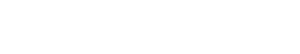# Statistics

Statistics refers to the study of the collection, analysis, interpretation, presentation, and organization of data. It deals with all aspects of this, including the planning of data collection in terms of the design of surveys and experiments.

Statistical theory is a branch of mathematics that deals with the analysis of random phenomena. Probability theory is a branch of mathematics that measures the likelihood that an event will occur. These two branches are closely related, and together they form the basis for statistical inference.

Statistical inference is the process of using data from a sample to make estimates or predictions about a population. This can be done in a number of ways, but the most common method is to use a statistical model.

A statistical model is a mathematical model that describes the underlying relationships between the variables in a data set. The most common type of statistical model is the linear regression model, which is used to predict a continuous outcome variable (such as income) from a set of predictor variables ( such as age, education, and gender).

Other types of statistical models include logistic regression, which is used to predict a binary outcome variable ( such as whether or not a person will vote), and Poisson regression, which is used to predict count data ( such as the number of accidents per year).

Statistical methods are used to help researchers design experiments, to analyze data from those experiments, and to make predictions about the results of future experiments.

There are many different types of statistical methods, and the choice of which method to use depends on the type of data, the research question, and the goals of the researcher. Some common statistical methods include:

• Descriptive statistics, which are used to summarize data
• Inferential statistics, which are used to make predictions about a population based on data from a sample
• Regression analysis, which is used to examine the relationships between variables
• ANOVA, which is used to compare means among groups
• Chi-square test, which is used to assess associations between categorical variables

Statistics is an important tool for text analytics because it allows us to understand and analyze data sets. It also allows us to make predictions about future events.Unlock the power of actionable insights with AI-based natural language processing.